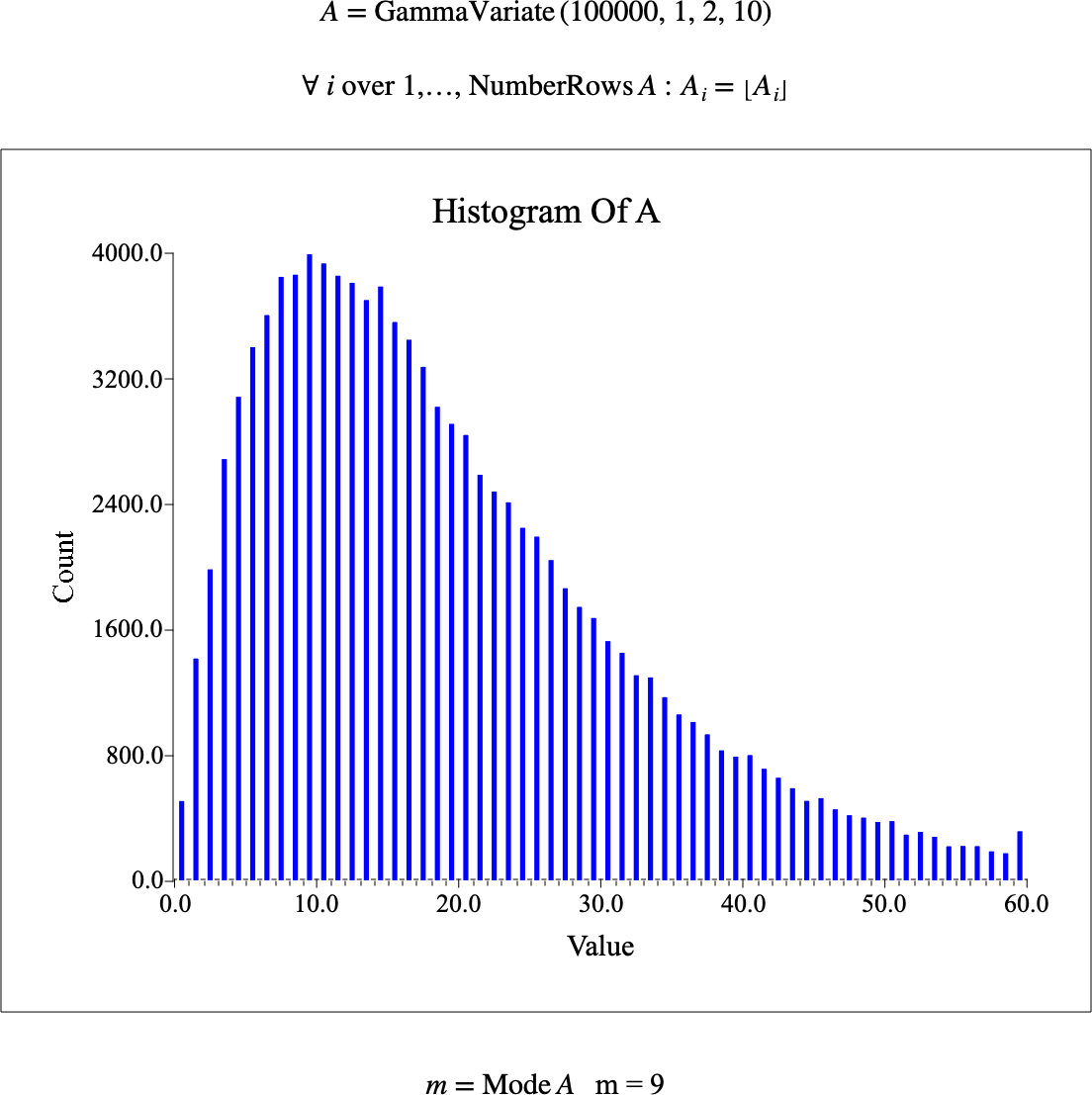# $$\text{Mode}$$¶

You can use the $$\text{Mode}$$ function to find the mode of a collection of scalar values.

You can use the \mode backslash command to insert this function.

The following variants of this function are available:

• $$\text{complex } \text{Mode} \left ( \ldots \right )$$

You can supply a list containing single values, sets, tuples, and matrices. If the supplied sets and tuples contain other sets and tuples, then this function will recursively locate the mode within those embedded iterable values.

Figure 176 shows the basic use of the $$\text{Mode}$$ function.Figure 176 Example Use Of The Mode Function